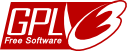# Simple C++ String Class

Every C++ programmer knows that the standard library has a string class. But, while learning, it’s a good idea to know how to develop your own string class.

That’s why I made the String class. It’s not intended for professional projects (for them, you should use the standard library’s string), but as help to learn C++.

```class String
{
char *m_pszString;	//Allocated buffer
int m_nAllocated;	//Allocated length

public:
//Construction and destruction
String() { /* ... */ }
~String() { /* ... */ }

//Copy constructors
String(const char *pszString) { /* ... */ }
String(const String& rsString) { /* ... */ }

//Operators (assignment)
String& operator=(const char *pszString);
String& operator=(const String& rsString) { /* ... */ }

//Operators (concatenation)
String& operator+=(const char *pszString);
String& operator+=(String& rsString) { /* ... */ }

//Operators (comparison)
bool operator<(String sString) { /* ... */ }
bool operator<=(String sString) { /* ... */ }

bool operator>(String sString) { /* ... */ }
bool operator>=(String sString) { /* ... */ }

bool operator==(String sString) { /* ... */ }
bool operator!=(String sString) { /* ... */ }

//Operations
void Clear();
String Lower() { /* ... */ }
String Upper() { /* ... */ }

//Information
int Length() { /* ... */ }

//Cast operators
operator const char*() { /* ... */ }

protected:	//Helper functions
/* ... */
};

//Output e input
```

This is a sample project made to explain the String class usage.

```#include <iostream>
using std::cout;
using std::cin;
using std::endl;
#include "String.h"

using namespace std;

int main(int argc, char *argv[])
{
cout << "String sample project" << endl;
cout << "---------------------" << endl << endl;

String a("This"), b("is"), c("a"), d("test");
String e = a + " " + b + " " + c + " " + d;

cout << "String parts:" << endl;
cout << '\t' << a << endl;
cout << '\t' << b << endl;
cout << '\t' << c << endl;
cout << '\t' << d << endl << endl;

cout << "Concatenated with spaces:" << endl;
cout << '\t' << e << endl << endl;

String f, g;

cout << "Please input a string (empty to break): ";
cin >> f;
cout << "Please input other string (empty to break): ";
cin >> g;

cout << endl;

cout << "1st string (" << f << ") is " << f.Length() << " chars long." << endl;
cout << "2nd string (" << g << ") is " << g.Length() << " chars long." << endl;

cout << endl;

cout << "1st string in lowercase is " << f.Lower() << endl;
cout << "1st string in uppercase is " << f.Upper() << endl << endl;

cout << "2nd string in lowercase is " << g.Lower() << endl;
cout << "2nd string in uppercase is " << g.Upper() << endl << endl;

cout << "1 < 2\t=> " << (f<g?"true":"false") << endl;
cout << "1 <= 2\t=> " << (f<=g?"true":"false") << endl;
cout << "1 > 2\t=> " << (f>g?"true":"false") << endl;
cout << "1 >= 2\t=> " << (f>=g?"true":"false") << endl;
cout << "1 == 2\t=> " << (f==g?"true":"false") << endl;
cout << "1 != 2\t=> " << (f!=g?"true":"false") << endl << endl;

if (f == g)
cout << "Strings are equal." << endl;
else
cout << "Strings are not equal." << endl;

cout << endl;

cout << "1+2\t=> " << f+g << endl;
cout << "2+1\t=> " << g+f << endl;

cout << endl;

return 0;
}
```

And this is its output:

```String sample project
---------------------

String parts:
This
is
a
test

Concatenated with spaces:
This is a test

Please input a string (empty to break): Hello, string 1
Please input other string (empty to break): Phrase 2

1st string (Hello, string 1) is 15 chars long.
2nd string (Phrase 2) is 8 chars long.

1st string in lowercase is hello, string 1
1st string in uppercase is HELLO, STRING 1

2nd string in lowercase is phrase 2
2nd string in uppercase is PHRASE 2

1 < 2   => false
1 <= 2  => false
1 > 2   => true
1 >= 2  => true
1 == 2  => false
1 != 2  => true

Strings are not equal.

1+2     => Hello, string 1Phrase 2
2+1     => Phrase 2Hello, string 1

```

The code completely is portable.

It’s been developed, compiled and tested using wxDev-C++ for Windows with the MinGW compiler (included in the bundle).String is licensed under the GNU GPL v3 (attached)…

### Support appreciated!

All the content offered in this website is, except noted otherwise, of free nature. This means you can share it wherever you want if you do it freely and stating its source.

If it was useful for you and you’d like to contribute, you can make a donation or, at least, visit one of our advertisers of your choice; they are all around the site.